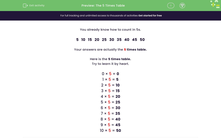# The 5 Times Table

In this worksheet, students answer questions on the 5 times table. Counting in 5s may help.Key stage:  KS 1

Curriculum topic:   Number: Multiplication and Division

Curriculum subtopic:   Solve Multiplication/Division Problems

Difficulty level:#### Worksheet Overview

You already know how to count in 5s.

5   10   15   20   25   30   35   40   45   50

Here is the 5 times table.

Try to learn it by heart.

0 × 5 = 0

1 × 5 = 5

2 × 5 = 10

3 × 5 = 15

4 × 5 = 20

5 × 5 = 25

6 × 5 = 30

7 × 5 = 35

8 × 5 = 40

9 × 5 = 45

10 × 5 = 50

### What is EdPlace?

We're your National Curriculum aligned online education content provider helping each child succeed in English, maths and science from year 1 to GCSE. With an EdPlace account you’ll be able to track and measure progress, helping each child achieve their best. We build confidence and attainment by personalising each child’s learning at a level that suits them.

Get started